For this one, let’s plug in. Let’s say Observer A observes an intensity of 16, so I = 16. Observer B would then observe an intensity of 1, so his I = 1.

Both observers are observing the same ratio signal, so the value of P will be the same for both. Because it won’t change, we can plug in for that, too. Let’s say P = 100. (You can really pick anything for this, but 100 is a good choice because you’ll end up taking its square root.)

Now solve both equations for r. I’m going to useand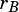to keep track of which distance is which.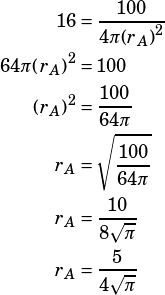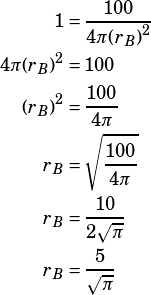The question asks for the ratio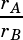. We can calculate that!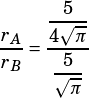That frankenfraction is not as gnarly as it looks. Just remember that dividing by a fraction is the same as multiplying by its reciprocal, and simplify:Heidi W says:Hsvwright says: